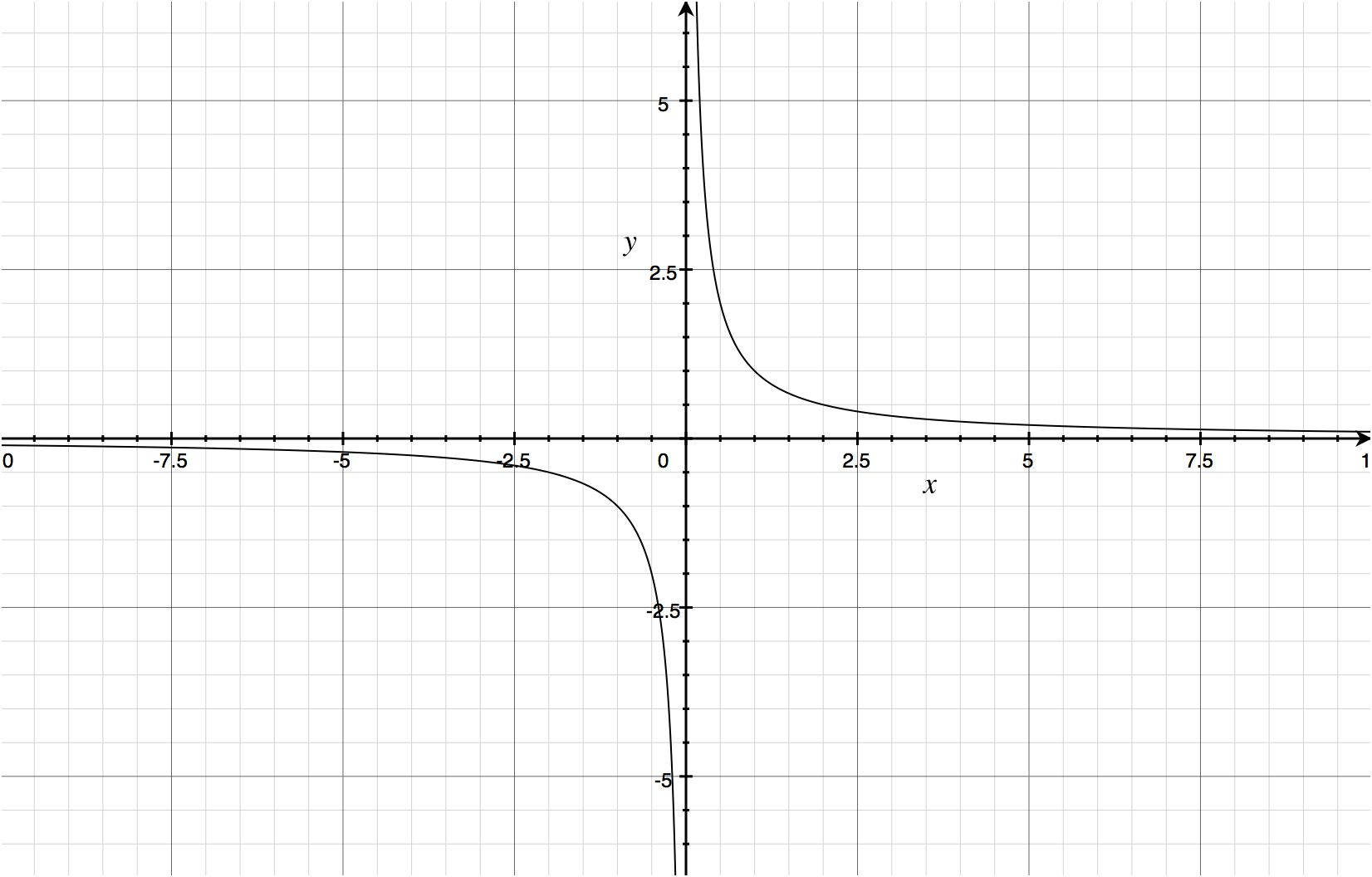# Is maths being taught incorrectly?

## 28 February 2014

We’ve all heard the complaints before: “I just don’t get maths!” “Oh no, not a maths problem! I failed that in school!” There is a pervasive fear and resentment of mathematics in westernized society, bordering on the level of derision that pop-culture takes toward religion.

I describe my own relationship with mathematics as being of a cold-war mode. Most of the time we’re cool with each other, maths and I, but there are times when what I really want to do is wring that sassy little textbook’s pages. I get that people have had bad experiences with maths, I truly do.

But perhaps the problem isn’t maths, but how it’s taught?

An example from my own life springs to mind, that is the (in)ability to divide by zero. We’re drilled from a young age that we simply can’t divide by zero. In my education, there was no explanation given. There was an instructor, an authority, and I was meant to accept that authority.

I don’t tend to do well with authority, at least not over long periods of time.

I recall badgering my Algebra teacher over it in the ninth grade, which in retrospect was a symptom of something being wrong. Since when does a freshman in high-school have the chutzpah to badger a teacher?

Poor Mrs. Reinke eventually gave up and told me that it approximates to infinity. (There was another fight a few months later as to whether an equals sign can be used to equate something divided by zero with infinity).

First, as I have later learned, it does not approximate to infinity. Consider the function

$f(x)=\frac{1}{x}.$

Let’s try taking the limit of this function as it approaches zero. A limit, in simple explanation, is trying to guess what a function will equal at a particular point in the domain by observing the values near that one. By taking the limit of this function, we should expect this to equal infinity based on what my maths teacher in the ninth grade said:

$\lim_{x\to 0}\frac{1}{x}.$

Only it doesn’t.From the graph, we can infer that $\lim_{x\to 0^{+}}\frac{1}{x}=\infty$ and $\lim_{x\to 0^{-}}\frac{1}{x}=-\infty$, which are indeed infinitely different. This is to say that if you’re approaching zero from the negative or left side of the domain, it’s equal to minus infinity; if you’re approaching zero from the positive or right side of the domain, it’s equal to positive infinity.

In mathematics, the definition of a function stipulates that any value in the domain must correspond to one and only one value in the range. Here it corresponds to two values in the range! (Assuming you consider infinity to be in the range, which it isn’t because infinity, both positive and negative variations, are not numbers - so it could just as easily be said that here zero corresponds to no values in the range)!

After much debate and probably a few deaths in the Pythagorean cult, mathematicians have decided that this kind of behavior is undefined because it doesn’t satisfy the definition of a function, ergo $\lim_{x\to 0}\frac{1}{x}$ is itself undefined.

Hence, we cannot divide by zero.

Back to maths education, a limit isn’t particularly hard to grasp on a conceptual level. The act of drawing the graph of $\frac{1}{x}$ shouldn’t escape even the most artistically-challenged teachers.

Why can’t we just show the graph and explain the concept when a student first asks the question “why can’t I divide by zero?” Instead of creating a veil of authority and mystique, trying to tell a student “you don’t know and you’re not smart enough to understand,” why can’t we simply teach?

I think this is a contributing factor in why people do not like maths today, which is altogether unfortunate. I believe that greater discourse in mathematics will yield social advances faster than a study of social advances will yield social advances.

If nothing else, someone will start to appreciate the corny maths jokes I like to tell at parties.